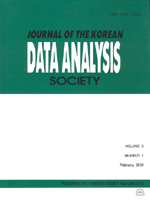상세검색
최근 검색어 전체 삭제
다국어입력
즐겨찾기0KCI등재 학술저널

# Cook-type Distance in Multivariate Constrained Regression

• 등재여부 : KCI등재
• 2009.04
• 629 - 636 (8 pages)

An influence measure, which we will call Cook-type distance, for multivariate regression with linear constraints on regression coefficients is suggested. It is a modification of the usual Cook’s distance adapted to multivariate constrained regression and it is defined by a distance between the estimates of regression coefficients evaluated with and without some observations, scaled by the covariance matrix of the distance, not by that of the estimates of regression coefficients as in the usual Cook’s distance. It would be used for detecting influential observations. Its sampling distribution will be derived, while that of the usual Cook’s distance is not available. Thus we can judge whether each observation is influential or not for multivariate constrained regression, while we can not use the cutoff values, based on the usual Cook’s distance, so that the use of the usual Cook’s distance is a rule of thumb. A numerical example is given for illustration.

1. Introduction

2. Preliminaries

3. Cook-type Distance

4. A numerical example

5. Concluding remarks

References